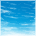## PWM read on HCS12 (Dragon 12)

cancel
Showing results for
Did you mean:

## PWM read on HCS12 (Dragon 12)

2,131 ViewsContributor I

I am having a problem reading a pwm signal into my HCS12. I am using codewarrior. I have been working on this problem for a long time with limited to no success. I am using input capture to time rising and falling edges on the samee channel(0). At this point my interupt is only firing on rising eges, but i think i have the register set correctly for both rising and falling. Any advice would be greatly appreciated.

Here is what i have:

#include <hidef.h>      /* common defines and macros */
#include <mc9s12dg256.h>     /* derivative information */
#include <stdio.h>
#include <stdlib.h>
#include <string.h>
#include <math.h>
#include "main_asm.h" /* interface to the assembly module */
#define DLC_DELAY 300
#define PI 3.14159265

__interrupt void RealTimeInterrupt( void );
__interrupt void TC0Interrupt( void );
__interrupt void TOI (void);

int diff,rise,fall,last,ovcnt,avg,sum,cnt;
char string;
int debug = 55,debug1=60;
int flag = 0;
double dblsum;

void main( void ){

PLL_init();
LCD_init();

DDRT= 0x00;                  //input

TIOS &= ~0x01;               // channel 0 input capture

TSCR1= 0x80;                 // enable timer

TIE = 1;                     // timer interupt enable

TSCR2= 0b101;                // prescale 32

TCTL4 = 0b11;                // capture any edge

EnableInterrupts;

while(1){

sum = sum + diff ;

if (cnt==100){

dblsum=sum ;
avg = dblsum/cnt;

cnt = 0;
sum = 0;
}

}

}

__interrupt void RealTimeInterrupt( void )
{
CRGFLG = 0x80;       /* clear rti flag */
}

__interrupt void TC0Interrupt( void )
{

TFLG1 = 0x01;     //clear TC0 flag

if(PTT &= 0x01){      //PT0 is high afte interupt
rise = TC0;

} else                   //PT0 is low afte interupt

{

fall = TC0;
diff = fall - rise;
cnt++ ;

}

}

__interrupt void TOI( void )
{

}

Labels (1)
• ### General

2 Replies
93 ViewsContributor I

I have made some progess. My averging was causeing some problems, so i bypassed it. I am getting very accurate readings on the low end of my desired scale (1ms-10ms), but around 10ms and above i am getting an occasional extermly high reading, as the hightime lengthens this error occurs more frequently.  Here's what i have now.

#include <hidef.h>      /* common defines and macros */
#include <mc9s12dg256.h>     /* derivative information */
#include <stdio.h>
#include <stdlib.h>
#include <string.h>
#include <math.h>
#include "main_asm.h" /* interface to the assembly module */
#define DLC_DELAY 300
#define PI 3.14159265

__interrupt void RealTimeInterrupt( void );
__interrupt void TC0Interrupt( void );
__interrupt void TOI( void );

volatile long unsigned int diff,rise,fall,last,ovcnt,sum=0,cnt,avg;
//float avg;
char string;
int debug = 1,debug2=2,debug3=3;
int flag = 0,flag2=0,flag3=0;
double dblsum;

void main( void ){

PLL_init();
LCD_init();
DDRT=0x00;                  //input

TSCR1=  0x80;                 // enable timer
TSCR2=  0b101;                // prescale 32
TIOS &= ~0x01;               // channel 0 input capture
TCTL4 =  0b11;                // capture any edge on channel 0
TIE =   1;                     // timer interupt enable

EnableInterrupts;

while(1){

if(flag==1){

sprintf(string,"%16d",debug);
writeLine(string, 1); //write to screen
flag=0;

}
if(flag2==1){

sprintf(string,"%16d",debug2);
writeLine(string, 1); //write to screen
flag2=0;

}

if(flag3==1){

sprintf(string,"%16d",debug3);
writeLine(string, 1);

flag3=0;

}

sum = sum + diff ;

if (cnt>10){

//avg = (float) (sum) / (float) (cnt);
avg = sum/cnt;
cnt = 0;
sum = 0;

sprintf(string,"%16lu",diff);
writeLine(string, 2); //write to screen
flag2=0;
}
}

}

__interrupt void RealTimeInterrupt( void )
{
CRGFLG = 0x80;       /* clear rti flag */
}

__interrupt void TC0Interrupt( void )
{

TFLG1 = 0x01;     //clear TC0 flag

if(PTIT & 0x01){

rise = TC0;
flag = 1;
} else

{

fall = TC0;
diff = fall - rise;

fall=0;
rise=0;

cnt++ ;

flag = 0;
flag2 = 1;

}

}

__interrupt void TOI( void )
{

flag2=0;
flag3 = 1;

}

93 ViewsContributor III

Hi,

My opinion: in TC0Interrupt routine, you may use PTIT register to

determine the level of input pin: if (PTIT & 0x01)...

Regards,

ipa